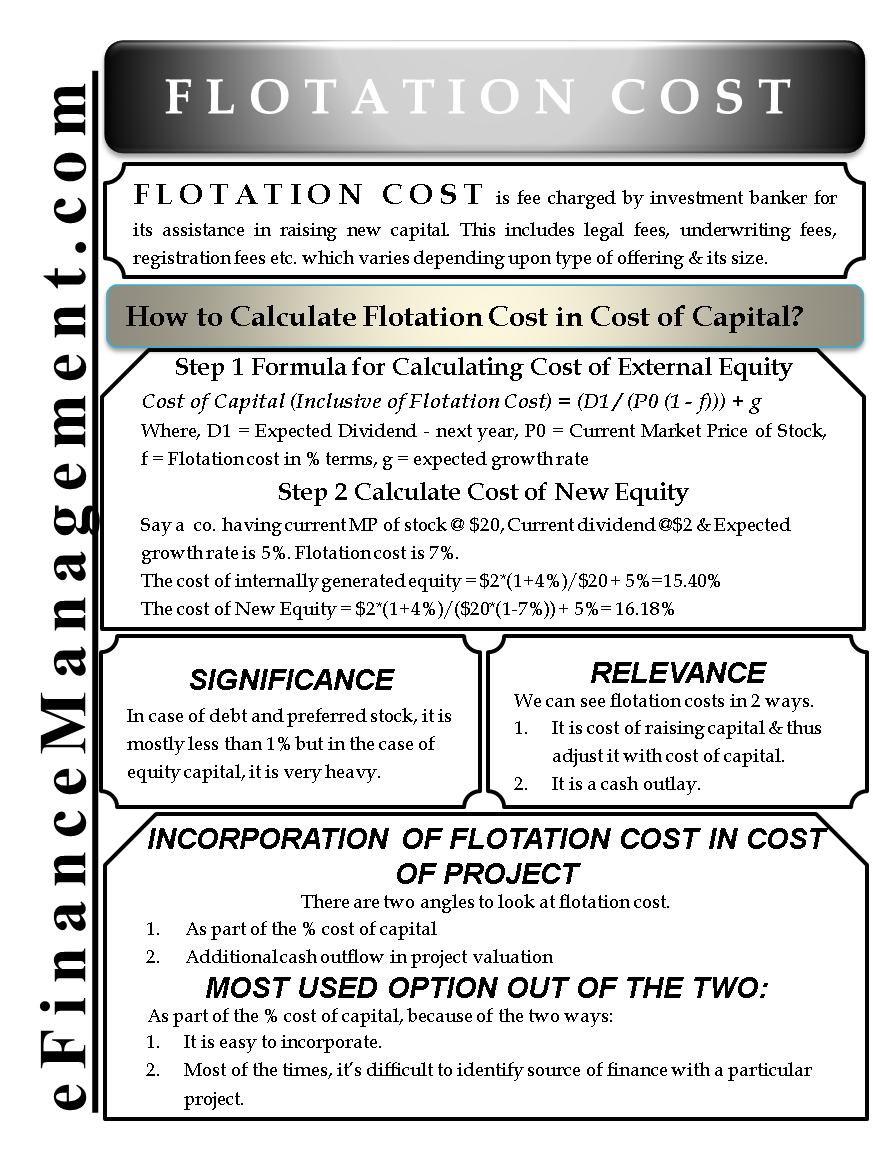data-full-width-responsive="true">

# Flotation Cost

## What is Flotation Cost in Finance?

Flotation cost is the fee charged by investment banker for its assistance in raising new capital. This flotation cost includes legal fees, underwriting fees, registration fees etc. The fee varies depending upon the type of offering and its size. This flotation cost is heavy in case of equity capital in comparison to debt and preferred stock. As a result, this cost has a significant relevance with equity issues. In fact, this is one of the many consideration before selecting new equity as a mode of financing.

## How significant is the Flotation Cost?

In the case of debt and preferred stock, it is mostly less than 1% but in the case of equity capital, it is very heavy. Average flotation cost in raising new equity in the US is around 7%, 2% in Germany, 6% in the UK, 5% in Swiss.

## How is Flotation Cost Relevant to the Corporation?

We pay the flotation cost in cash at the time of issuance of equity shares. It is a cost that has to be incorporated into the project/projects of the company to arrive at the right profitability. This cost has to be a part of the evaluation of projects. We can see it in 2 ways.

1. It is the cost of raising the capital and therefore adjust it with the cost of capital.
2. It is a cash outlay like the other initial cash outlay of the project.

In essence, while calculating the net present value of a project, either we adjust it with the numerators (Cash inflow or outflow) or with the denominators (discounting rate).## How to Calculate Flotation Cost in Cost of Capital?

Let’s check out step by step.

### Formula for Calculating Cost of External Equity

Cost of Capital (Inclusive of Flotation Cost) = (D1 / (P0 (1 – f))) + g

Where, D1 = Expected Dividend – next year, P0 = Current Market Price of Stock, f = Flotation cost in % terms, g = expected growth rate

### Calculate Cost of New Equity

Suppose a company having a current market price of stock @ \$20, current dividend @\$2 and its expected growth rate is 5%. Flotation cost is 7%.

The cost of internally generated equity = \$2*(1+4%)/\$20 + 5%=15.40%

The cost of New Equity = \$2*(1+4%)/(\$20*(1-7%)) + 5%= 16.18%

Flotation cost in Cost of Capital = 16.18-15.40 = 0.78 ~ 78 basis points in New Equity Cost of Capital.

## How to Incorporate Flotation Cost in the Cost of Project?

There are two angles to look at flotation cost.

1. As part of the % cost of capital
2. Additional cash outflow in project valuation

Let’s further assume the company is entering a new project which requires a total capital of \$100000. The company will finance it 40% by debt of 6% and 60% by Equity. The tax rate is 40%. Assume inflow from the project is \$20000 every year till 15 years.

Let’s now calculate the NPV of the project. For calculating the NPV, we will need the WACC for the same.

 Source of Capital New Issuance Proportion Cost of Capital Cost of Capital (Absolute) Cost of Capital (after Flotation Cost) Cost of Capital (Absolute) Debt 40,000.00 0.40 3.60% 1,440.00 3.60% 1,440.00 Equity 60,000.00 0.60 15.40% 9,240.00 16.18% 9,708.00 WACC 1,00,000.00 1.00 10.68% 10,680.00 11.15% 11,148.00

Now, we need to calculate the NPV for the project for both the situation.

### Part of the Cost of Capital

WACC = 11.15% when the flotation cost is part of the cost of capital.

When flotation cost is part of cost of capital, NPV = 117057 – 100000 = 17057

### Additional Cash Outflow in Project Valuation

WACC = 10.68% when the flotation cost is part of the cash flows.

When flotation cost is part of cash flows, NPV = 119382 – 100000 – 60000*7% = 19382 – 4200 = 15182

We notice that there is a difference in calculation between the two approaches. It is more appropriate to deduct the flotation cost from the NPV calculation. By affecting the cost of capital by 78 basis points, we are giving an effect of that on all the future cash flows. If we look otherwise, the flotation cost is an initial outlay because it was never received by the company.

On the contrary, in most places, the effect of flotation cost is given in the cost of capital. There are primarily two reasons for that.

1. It is easy to incorporate.
2. Most of the times, it is difficult to identify the source of finance with a particular project.
Last updated on : November 12th, 2018

** Disclaimer: This post may contain Affiliate Links marked as ** and we may earn a commission on sale.

What’s your view on this? Share it in comments below.